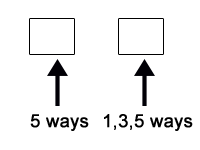# Sample Aptitude Questions of Intergraph

INTERGRAPH Aptitude Questions
1. The value of (1.073*1.073 - 0.927*0.927 / 1.073 - 0.927 ) +  (34)4 *96 / (27)7*(3)9 is
1. 2
2. 1/9
3. 2 1⁄9
4. 3 1⁄9

Solving this expression, we get:
=> (1.073)2 - (0.927 ) 2/ 1.073 - 0.927 ) + (3)16 *(32)6 / (33)7*(3)9
=> (1.073 -0.927)(1.073+0.927) / (1.073 -0.927) + (3)16 *(3)12 / (3)21*(3)9
=> (1.073+0.927) + (3)28 / (3)30
=> 2 + 1/3= 2 + 1/9 = 19/9 = 2 1⁄9
We get the answer as C option.
2. The odds against a certain event are 5 : 2 and the odds in favour of another independent event are 6 : 5. The probability that at least one of the events will happen is:
1. 12/77
2. 25/77
3. 52/77
4. 65/77

Required probability = 1 – P (NO event will happen) = 1 – (5/7 × 5/11) = 52/77.
1. Number of times the hands of a clock are in a straight line every day is:
1. 44
2. 24
3. 42
4. 22

In 12 hour, the hands of clock coincide 11 times and they make 180° also 11 times, thus total of 22 times. So in 24 hours, the hands of clock will be forming a straight line 22 + 22 = 44 times.
2. A motor boat can travel at 10 km/h in still water. It traveled 91 km downstream in river and then returned, taking altogether 20 hours. Find the rate of flow of river.
1. 3 kmph
2. 5 kmph
3. 6 kmph
4. 7 kmph

Let the rate of flow be y kmph. The equation that will be formed is 91/(10+y) + 91/(10-y) = 20
Solving we get y = 3.
3. If x4 + 1/x4 = 47, find the value of x3 + 1/x3.
1. 18
2. 20
3. 22
4. 24

x4 + 1/x4 + 2 = 47 + 2 => x2 + 1/x2 = 7;
Also,x2 + 1/x2 + 2 =  9 => x+1/x = 3
Now,( x+1/x)=x3 + 1/x3 + 3( x+1/x)
=> 27 = x3 + 1/x3 + 3(3)
=>x3 + 1/x3 =  18.
4. A person invests Rs. 5508 in '4% stock at 102'. He afterwards sells out at 105 and reinvests in '5% stock at 126'. What is the change in his income?
1. Rs. 20
2. Rs. 7
3. Rs. 10
4. Rs. 9

Old income = 5508/102 × 4 = Rs. 216. No. of shares purchased = 5508/102 = 54. So sale proceeds = (54 × 105) = Rs. 5670.
Therefore, New income = (5670 × 5)/126 = Rs. 225.
So change in income = 225 – 216 = Rs. 9.
5. The ratio between the number of sides of two regular polygons is 1 : 2 and the ratio between the measure of their interior angles is 2 : 3. The number of sides of these polygons are respectively:
1. 4, 8
2. 5, 10
3. 6, 12
4. 8, 16

Going by options, if the no of sides are 4 and 8, then the interior angles of the polygons is 90° and 135° (int angles = 180°(N-2)/N)
Which are in the ratio 2 : 3. So the answer is 1st option.
6. How many two-digit odd numbers can be formed from the digits 1, 2, 3, 4, 5, if repetition of digit is allowed?
1. 5
2. 15
3. 35
4. 25Here unit place can be filled in 3 ways (i.e. 1, 3, 5)
Ten's place can be filled in 5 ways. Required number of numbers = 5×3 = 15
7. Three equal glasses are filled with mixtures of sprit and water. The ratio of the sprit to water is as follows: in the first glass as 3 : 4, in the second glass as 4 : 5 and in the third glass as 5 : 6.The contents of the three glasses are emptied into a single vessel. What is the ratio of the sprit to water in the mixture now?
1. 920 : 1159
2. 820 : 1149
3. 1120 : 1134
4. 1010 : 1122